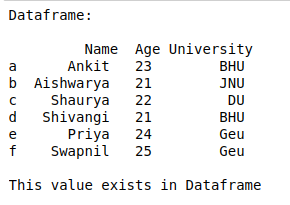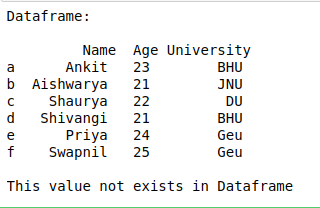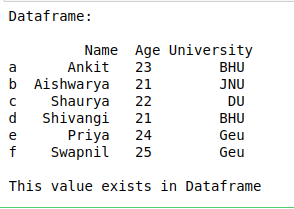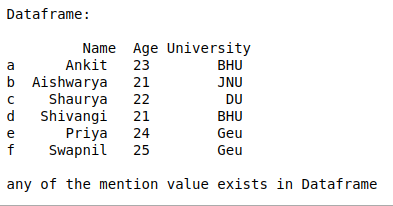# Check if a value exists in a DataFrame using in & not in operator in Python-Pandas

In this article, Let’s discuss how to check if a given value exists in the dataframe or not.
Method 1 : Use in operator to check if an element exists in dataframe.

## Python3

 `# import pandas library` `import` `pandas as pd`   `# dictionary with list object in values` `details ``=` `{` `    ``'Name'` `: [``'Ankit'``, ``'Aishwarya'``, ``'Shaurya'``, ` `              ``'Shivangi'``, ``'Priya'``, ``'Swapnil'``],` `    ``'Age'` `: [``23``, ``21``, ``22``, ``21``, ``24``, ``25``],` `    ``'University'` `: [``'BHU'``, ``'JNU'``, ``'DU'``, ``'BHU'``, ``'Geu'``, ``'Geu'``],` `}`   `# creating a Dataframe object ` `df ``=` `pd.DataFrame(details, columns ``=` `[``'Name'``, ``'Age'``, ``'University'``],` `                  ``index ``=` `[``'a'``, ``'b'``, ``'c'``, ``'d'``, ``'e'``, ``'f'``])`   `print``(``"Dataframe: \n\n"``, df)`   `# check 'Ankit' exist in dataframe or not` `if` `'Ankit'` `in` `df.values :` `    ``print``(``"\nThis value exists in Dataframe"``)`   `else` `:` `    ``print``(``"\nThis value does not exists in Dataframe"``)`

Output :Method 2: Use not in operator to check if an element doesn’t exists in dataframe.

## Python3

 `# import pandas library` `import` `pandas as pd`   `# dictionary with list object in values` `details ``=` `{` `    ``'Name'` `: [``'Ankit'``, ``'Aishwarya'``, ``'Shaurya'``, ``'Shivangi'``, ``'Priya'``, ``'Swapnil'``],` `    ``'Age'` `: [``23``, ``21``, ``22``, ``21``, ``24``, ``25``],` `    ``'University'` `: [``'BHU'``, ``'JNU'``, ``'DU'``, ``'BHU'``, ``'Geu'``, ``'Geu'``],` `}`   `# creating a Dataframe object ` `df ``=` `pd.DataFrame(details, columns ``=` `[``'Name'``, ``'Age'``, ``'University'``],` `                  ``index ``=` `[``'a'``, ``'b'``, ``'c'``, ``'d'``, ``'e'``, ``'f'``])`   `print``(``"Dataframe: \n\n"``, df)`   `# check 'Ankit' exist in dataframe or not` `if` `'Ankita'` `not` `in` `df.values :` `    ``print``(``"\nThis value not exists in Dataframe"``)`   `else` `:` `    ``print``(``"\nThis value exists in Dataframe"``)` `   `

Output :Method 3 : Check if a single element exist in Dataframe using isin() method of dataframe.

## Python3

 `# import pandas library` `import` `pandas as pd`   `# dictionary with list object in values` `details ``=` `{` `    ``'Name'` `: [``'Ankit'``, ``'Aishwarya'``, ``'Shaurya'``, ``'Shivangi'``, ``'Priya'``, ``'Swapnil'``],` `    ``'Age'` `: [``23``, ``21``, ``22``, ``21``, ``24``, ``25``],` `    ``'University'` `: [``'BHU'``, ``'JNU'``, ``'DU'``, ``'BHU'``, ``'Geu'``, ``'Geu'``],` `}`   `# creating a Dataframe object ` `df ``=` `pd.DataFrame(details, columns ``=` `[``'Name'``, ``'Age'``, ``'University'``],` `                  ``index ``=` `[``'a'``, ``'b'``, ``'c'``, ``'d'``, ``'e'``, ``'f'``])`   `print``(``"Dataframe: \n\n"``, df)`   `# isin() methods return Boolean ` `# Dataframe of given Dimension` `# first any() will return boolean series` `# and 2nd any() will return single bool value` `res ``=` `df.isin([``'Ankit'``]).``any``().``any``()`   `if` `res :` `    ``print``(``"\nThis value exists in Dataframe"``)`   `else` `:` `    ``print``(``"\nThis value does not exists in Dataframe"``)`

Output :Method 4 : Check if any of the given values exists in the Dataframe using isin() method of dataframe.

## Python3

 `# import pandas library` `import` `pandas as pd`   `# dictionary with list object in values` `details ``=` `{` `    ``'Name'` `: [``'Ankit'``, ``'Aishwarya'``, ``'Shaurya'``, ``'Shivangi'``, ``'Priya'``, ``'Swapnil'``],` `    ``'Age'` `: [``23``, ``21``, ``22``, ``21``, ``24``, ``25``],` `    ``'University'` `: [``'BHU'``, ``'JNU'``, ``'DU'``, ``'BHU'``, ``'Geu'``, ``'Geu'``],` `}`   `# creating a Dataframe object ` `df ``=` `pd.DataFrame(details, columns ``=` `[``'Name'``, ``'Age'``, ``'University'``],` `                  ``index ``=` `[``'a'``, ``'b'``, ``'c'``, ``'d'``, ``'e'``, ``'f'``])`   `print``(``"Dataframe: \n\n"``, df)`   `# isin() methods return Boolean Dataframe` `# of given Dimension first any() will return` `# boolean series and 2nd any() will return ` `# single boolean value` `res ``=` `df.isin([``'Ankit'``, ``'good'``, ``30``]).``any``().``any``()`   `if` `res :` `    ``print``(``"\nany of the mention value exists in Dataframe"``)` `    `  `else` `:` `    ``print``(``"\nNone of these values exists in Dataframe"``)`

Output :Whether you're preparing for your first job interview or aiming to upskill in this ever-evolving tech landscape, GeeksforGeeks Courses are your key to success. We provide top-quality content at affordable prices, all geared towards accelerating your growth in a time-bound manner. Join the millions we've already empowered, and we're here to do the same for you. Don't miss out - check it out now!